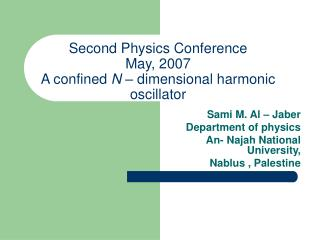# Second Physics Conference May, 2007 A confined N – dimensional harmonic oscillator - PowerPoint PPT PresentationDownload PresentationSecond Physics Conference May, 2007 A confined N – dimensional harmonic oscillator

Second Physics Conference May, 2007 A confined N – dimensional harmonic oscillatorDownload Presentation## Second Physics Conference May, 2007 A confined N – dimensional harmonic oscillator

- - - - - - - - - - - - - - - - - - - - - - - - - - - E N D - - - - - - - - - - - - - - - - - - - - - - - - - - -
##### Presentation Transcript

1. Second Physics ConferenceMay, 2007A confined N – dimensional harmonic oscillator Sami M. Al – Jaber Department of physics An- Najah National University, Nablus , Palestine

2. Abstract • We compute the energy eigenvalues for the N- dimensional harmonic oscillator confined in an impenetrable spherical cavity. The results show their dependence on the size of the cavity and the space dimension N. The obtained results are compared with those for the free N- dimensional harmonic oscillator, and as a result, the notion of fractional dimensions is pointed out. Finally, we examine the correlation between eigenenergies for confined oscillators in different dimensions.

3. 2- The N- dimensional harmonic oscillator • The radial part solution, , satisfies

4. lettingR (r)=u (r) / r(N-1)/2 and Looking for solutions of the

5. We get And thus

6. 1F1(a,b,z)= • Where,

7. Connection between a confined harmonic oscillator and a free one • The value of λ is • We consider the case a = -1and

8. Table 1: a = -1 and

9. Table 2: The case a = -1 and

10. For a = -2, 1F1(a,b,z) has two roots. and • For the ground state we have and b =N /2, and thus • The two roots z1 and z2 yield two radii for the cavity , S1 and S2, which correspond to the states = ( 4 ,0) and ( 4 , 2) for the free harmonic oscillator

11. Table 3: a = -2 and

12. General Case • = even integer implies even or odd. The n = even case yields mod 4, a = negative integer, and this energy corresponds to the states for the free harmonic oscillator with whose number is n /2.

13. n= odd case yields mod 4, a = negative half – integer, and the energy corresponds to the states for the free harmonic oscillator with whose number is (n+1)/2. n = odd integer : using n =half odd integer. This energy could be written in two ways: The first: which is the same as that of the states of the free harmonic oscillator in (N-1) dimensions. The values of are given by

14. The second: which is the same as that of the states of the free harmonic oscillator in(N+1) dimensions. The values of are given by

15. defined

16. For computational purposes, we choosec=1 and and it is just the energy of the state ( 0 ,0) or ( 1 , 1) for the free harmonic oscillator in ( N+1) or ( N-1) dimensions respectively,

17. Table 5. c = 1

18. c=half odd integer: For example if c= , then the energy of the confined oscillator becomes This energy could be written as which is just the ground – state energy of the state (n' , ) (0 ,0 ) for the free harmonic oscillator in the fractional dimension if we let with n being odd, then the energy of the confined harmonic oscillator becomes This energy could be written as which is exactly the energy of the state (n' , )= for the free harmonic oscillator in the fractional dimension

19. 4- Energy levels and eigenfunctions for a confined harmonic oscillator Letting the value of, for a given at which 1F1 has its zero at We numerically compute E10, E21, and E32 for two cavities.

20. Table 6: computed energy levels

21. Table 7:Results for E10 & E20 for S=4A0.

22. It is observed thatE10 corresponds to the state (n , ) = (0 , 0) for the free N –dimensional harmonic oscillator.E20 corresponds to the state ( 2, 0) with energy • One also observes that the effect of the boundary is relatively larger on the energy levelE20 compared to its effect onE10. • Mapping of energy eigenvalues For example, the energies for in dimension N =5 are identical to the three- dimensional solutions for the case

23. ForN = odd and angular momentum the energies correspond to solutions for the roots of 1F1 • These correspond exactly to those either for dimension (N-2) and angular momentum or they correspond to the three dimensional case with • For N = even and angular momentum the energies correspond to those in dimension (N-2) with angular momentum or they correspond to those for two- dimensional case (N=2) with

24. Conclusion • The energy eigenfunctions were computed numerically using mathematica. • We pointed out the connection between solutions for the confined oscillator and the free one. • The effect of the boundaries and the dimension N were discussed. • The notion of fractional dimensions was explored. • Mapping between energy eigenvalues in different dimensions was examined.